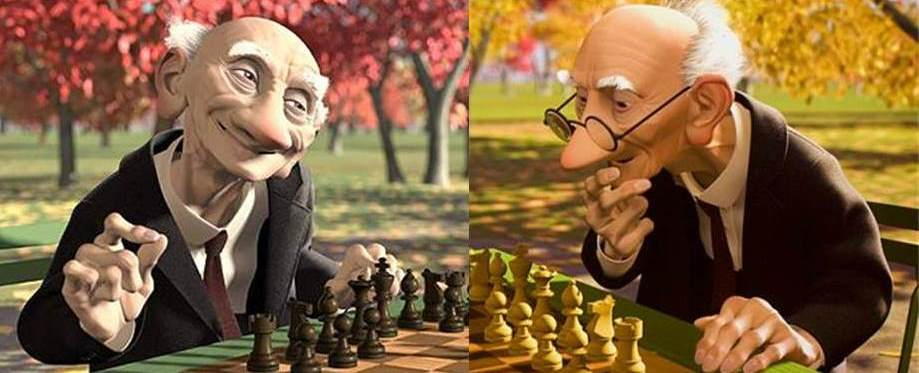# Two Math ProfessorsTwo math professors, professor Uno and professor Dos, play chess at the park while reminiscing about their past.

Prof. Uno say, "It just crossed my mind that when we first met, the square of your age contains the same three digits as the square of my age but in a different order."

Prof. Dos reply, "If you take the square of the sum of our ages when we first met and split it into two 2-digit numbers, you will have my age then and your age now."

If the sum of their current ages can be expressed as a 3-digit number $\overline{abc}$, then calculate $a^3+b^3+c^3$.

×